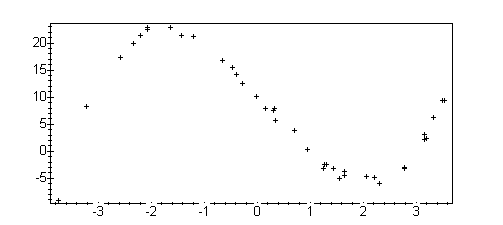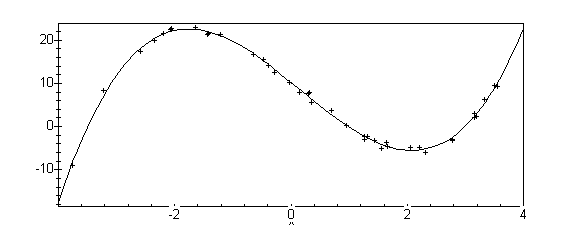## Fitting a cubic to data

Let's now try to fit a cubic polynomial to some data. Again, maple's built-in package can do this for us, but we like to work harder than we need to, so we understand more. First, let's get some data, using the provided function

```cubdata := read(`/home/mat331/lsq_data`):
plot(cubdata,style=point, axes=boxed);
```As in the linear case, we will do the work "by hand".

To simplify typing, let's define the type of function we want to fit. We know (somehow) that our goal is to fit a cubic polynomial, so

``` cub := (x,a,b,c,d) ->  a*x^3 + b*x^2 + c*x + d;

3      2
cub := (x, a, b, c, d) -> a x  + b x  + c x + d
```

Now we define an "error functional" which gives us the distance from our cubic to our data. Note that we use maple's "nops" command so that we don't need to explicitly say how many points there are.

```G := (a,b,c,d) -> sum((cubdata(pts[i],a,b,c,d) - cubdata[i] )^2,
i=1..nops(cubdata));

nops(cubdata)
-----
\                                                    2
G := (a, b, c) ->     )     (cub(cubdata[i], a, b, c,d) - cubdat[i])
/
-----
i = 1
```

Now we just ask maple to find the (unique) critical point of G, which is our minimum. Since we used assign to set the coefficients, we won't see a result.

```assign(solve( {diff( G(a,b,c,d), a)=0,
diff( G(a,b,c,d), b)=0,
diff( G(a,b,c,d), c)=0},
diff( G(a,b,c,d), d)=0},
{a,b,c,d} ));
```

```cub(x,a,b,c,d);

3                2
x  - .4806519894 x  - 10.94459406 x + 10.28284644
```

Finally, lets make a picture, just to see how well it fits

```with(plots):
cplot := plot(cub(x,a,b,c,d), x=-4..4, axes=boxed):
pplot:= plot(cubdata, style=point):
display({cplot, pplot});
```Just for comparison, we can let maple do this for us, using the built-in least-squares package:

``` with(stats):
fit[leastsquare[[x,y],y=A*x^3+B*x^2+C*x+D]]
([[cubdata[i]\$i=1..nops(cubdata)],
[cubdata[i] \$i=1..nops(cubdata)]]);

3                2
y =    x  - .4806519892 x  - 10.94459410 x + 10.28284642
```